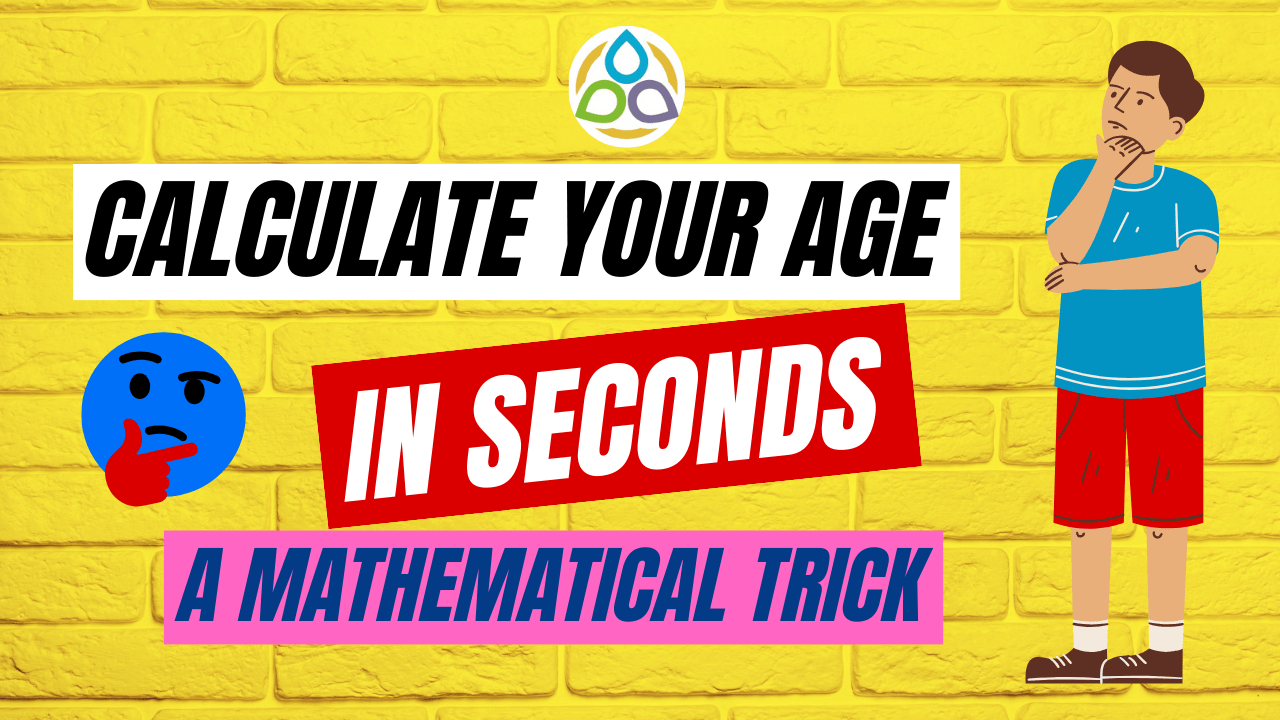## Calculate Your Age in Seconds – A Mathematical Trick

Algebra serves as a remarkable tool for determining the unknown age of an individual through a series of mathematical operations. This intriguing process unfolds like magic, enabling anyone to deduce their age without disclosing the information to others. By engaging in specific algebraic calculations, participants in this game can arrive at a final result. With this conclusive value in hand, I, as an observer, can effortlessly ascertain the person’s age when presented with the obtained outcome. Let’s explore this concept further through the following example to gain a better understanding.

Let’s consider the age of a person to be 7 (written as 07) years, where the first digit is 0 and the second digit is 7. To perform the given operations:

• 1. Multiply the first digit of the age by 5: 0 × 5 = 0
• 2. Add 3 to the result: 0 + 3 = 3
• 3. Double the answer: 3 × 2 = 6
• 4. Add the second digit of the age to the doubled result: 7 + 6 = 13
• 5. Subtract 6 from the sum: 13 – 6 = 7

After performing the specified operations, the final result is 7, which corresponds to the provided age.

Below is a mathematical trick presented for calculating the age of individuals using algebraic methods.

• 1.      Multiply the first digit (or number) of the age by 5.
• 2.      Add 3 to the result.
• 4.      Add the second digit of the number with the result.
• 5.      Subtract 6 from it.
• 6.      The result is your age!

Now consider another example.

Let the age of the person be 102 years. Since it is a three-digit number, we consider 10 as the first number and the second digit of the age is 2. Multiplying the first digit of the age by 5, we get 50. Now adding 3 results in 53. Doubling this answer yields 106. Next, adding the second digit of the age to this result, we get 2 + 106 = 108 (as the second digit of the age is 2). Subtracting 6 from it, we get 108 – 6 = 102. Therefore, the calculated age is 102, matching the given age of the person.

Here is a rigorous proof of this result using algebra:

Proof. In this proof, we will employ algebra to demonstrate the universality of the given result.

Case I. Assume that the ages we are considering are integers less than 100. We can represent it as

xy = 10x + y, where 0 ≤ x ≤ 9 and 0 ≤ y ≤ 9.

Here, x is the first (or ten’s) digit of the age, and y is the second (or unit) digit of the age.

1.      Multiplying the first digit of the age by 5 gives 5 × x = 5x

2.      Adding 3 to the result yields (5x + 3).

3.      Doubling the answer gives 2 × (5x + 3).

4.      Adding the second digit of the age with the result yields

5.      Subtracting 6 from it, we finally obtain

6.      The result is the age xy!

Case II. Assume that the ages we are considering are integers greater than or equal to 100. We can represent it as

xy = 10x + y, where x ≥ 10 and 0 ≤ y ≤ 9.

Here, x is the first number of the age, and y is the second digit of the age. We can demonstrate that the result also holds in this case.

It follows that, no matter what values of x and y we choose, the above steps will consistently yield our age.

Do you have any other age calculation tricks in mind that you would like to see in the next blog post? Feel free to let us know.

Would you like to write an outstanding non-routine article and get published on Math1089? Whether you have an idea for unparalleled content for the next blog post or you want to contribute an outstanding article, please let us know your ideas.”

This blog is as much yours as it is mine. So, if you have got some ideas to share what you want to see in the next post, feel free to drop a line. We welcome your ideas with open arms and reverence! Looking forward to seeing you soon on “Math1089 Mathematics for All” for another fascinating mathematics blog.